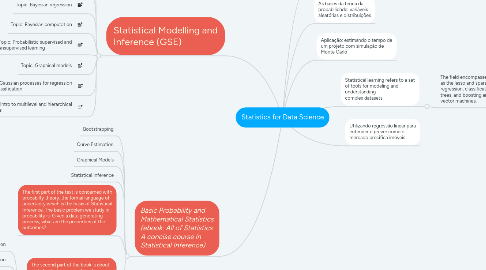# Statistics for Data ScienceRicardo (Xbalieiro)
Começar. É Gratuito
ou inscrever-se com seu endereço de e-mailStatistics for Data Science## 7. Basic Probability and Mathematical Statistics (ebook: All of Statistics A concise course in Statistical Inference)

### 7.6. The second part of the book is about statistical inference and its close cousins, data mining and machine learning. The basic problem of the statistical inference is the inverse of probability: Given the outcomes, what can we say about the process that generated the data?

7.6.1. Prediction

7.6.2. Classification

7.6.3. Clustering

7.6.4. Estimation

7.6.5. Data Analysis, Machine Learning and Data mining are various names given to the practive of Statistical Inference, depending on the context.